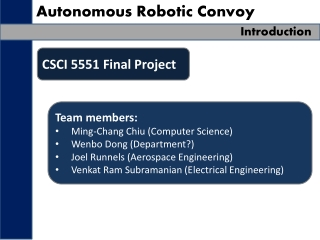# Autonomous Robotic Convoy - PowerPoint PPT PresentationDownload PresentationAutonomous Robotic Convoy

Autonomous Robotic Convoy
Download Presentation## Autonomous Robotic Convoy

- - - - - - - - - - - - - - - - - - - - - - - - - - - E N D - - - - - - - - - - - - - - - - - - - - - - - - - - -
##### Presentation Transcript

1. Autonomous Robotic Convoy Introduction CSCI 5551 Final Project • Team members: • Ming-Chang Chiu (Computer Science) • WenboDong (Department?) • Joel Runnels (Aerospace Engineering) • Venkat Ram Subramanian (Electrical Engineering)

2. Autonomous Robotic Convoy Motivation “Road trains” are a series of freight trailers pulled by a single tractor. • Advantages: • Decreased drag leads to higher efficiency • Only one driver required to move several truck-loads of freight • Disadvantages: • Difficult to maneuver • Accident prone • Limited to use on low-traffic routes

3. Autonomous Robotic Convoy Motivation • Most problems caused by the dynamic coupling between multiple vehicles • Each additional car introduces another degree of freedom • More degrees of freedom leads to a more chaotic system • Each additional car makes the system harder to control in sub-optimal conditions

4. Autonomous Robotic Convoy Motivation • Robotic convoys avoid these problems • Robotic convoys rely on sensors to follow the next car in the train • No mechanic coupling between cars means no complex dynamics • Each unit in convoy would be able to detect dangerous situations and avoid halt maneuvers which could lead to accidents

5. Autonomous Robotic Convoy Assumption Initial range of leader is known Initial distance to the is also known Only one person is moving at first

6. Autonomous Robotic Convoy Previous Work INSERT LITERATURE REVIEW HERE!

7. Autonomous Robotic Convoy Problem Description Problem Statement: Develop a prototype robotic convoy system • Assumptions: • Each unit in the robotic convoy will follow a “leader” on an arbitrary path • Workspace of robot convoy is not “clean,” i.e. the robots must be able to discern between leader and other obstacles (stationary or moving) • Workspace assumed to be flat, i.e. no obstacles or “pot holes” in workspace that are undetectable by robots • Equipment: • Pioneer 3 Robots by Adept MobileRobots • SICK laser scanner

8. Autonomous Robotic Convoy Methodology Robotic Convoy: Two main tasks Object Detection Path Planning

9. Autonomous Robotic Convoy Methodology Workflow: Identify Leader Detect Objects Plan Path Execute Path

10. Autonomous Robotic Convoy Methodology Object Detection • SICK laser scanner gives distance as a function of angle, • Software calculates “derivative” INSERT PLOTS FROM SICK LASER SCANNER HERE!

11. Autonomous Robotic Convoy Methodology Object Detection • Objects identified by signature peaks in “derivative” • Software identifies object “center” angle and distance INSERT PLOTS FROM SICK LASER SCANNER HERE!

12. Autonomous Robotic Convoy Methodology Object Velocity Measurement • Two data-sets taken, separated by specified time (0.1 seconds) Object B (t) Object B (t + 0.1) Object C (t) Object C (t + 0.1) Object A (t) Object A (t + 0.1) Robot

13. Autonomous Robotic Convoy Methodology Object Velocity Measurement Robot must be able to identify which object at corresponds to a given object at Object ? (t) Object C (t) Object ? (t + 0.1) Object ? (t + 0.1) Object ? (t + 0.1) Object ? (t) Robot

14. Autonomous Robotic Convoy Methodology Object Velocity Measurement Software calculates the “potential velocity” needed for an object at to make it to each object position at Object ? (t + 0.1) Object ? (t + 0.1) Object ? (t + 0.1) Object ? (t) Robot

15. Autonomous Robotic Convoy Methodology Object Velocity Measurement Software assumes that lowest “potential velocity” is the correct velocity. Object ? (t + 0.1) Object ? (t + 0.1) Object ? (t + 0.1) Object ? (t) Robot

16. Autonomous Robotic Convoy Methodology Path Planning Following Turning • Give a tolerance degree(theta), • If object exceeds the theta, then check if the robot still can see the object, if it can, then just follow, else turn

17. Autonomous Robotic Convoy Results INSERT RESULTS SLIDES HERE!

18. Autonomous Robotic Convoy Future Work • Immediate Future Work: • Continue development and implementation of path-planning and execution • Develop more robust obstacle avoidance methods • Test software in varied environments • Long-Term Future Work: • Implement inter-robot communication using wireless transmitters • Implement additional sensors which can detect more subtle obstacles • Implement absolute location measurement (GPS)

19. Autonomous Robotic Convoy Conclusion • Algorithms for robotic convoy under continual development • Object detection algorithms have been tested and implemented on Pioneer 3 units • Path planning algorithms still under development and continual testing

20. Autonomous Robotic Convoy Conclusion Thank You!